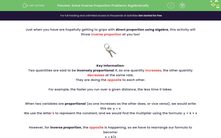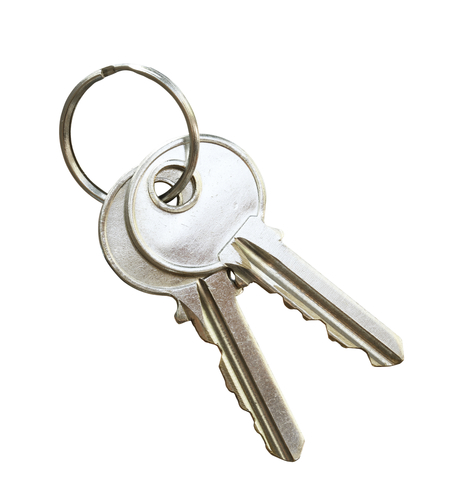# Solve Inverse Proportion Problems Algebraically

In this worksheet, students will find the values of unknown variables which are inversely proportional to each other, using appropriate notation to express such relationships algebraically.Key stage:  KS 4

Year:  GCSE

GCSE Subjects:   Maths

GCSE Boards:   AQA, Eduqas, Pearson Edexcel, OCR,

Curriculum topic:   Ratio, Proportion and Rates of Change

Curriculum subtopic:   Ratio, Proportion and Rates of Change Direct and Inverse Proportion

Difficulty level:#### Worksheet Overview

Just when you have are hopefully getting to grips with direct proportion using algebra, this activity will throw inverse proportion at you too!Key Information:

Two quantities are said to be inversely proportional if, as one quantity increases, the other quantity decreases at the same rate.

They are doing the opposite to each other.

For example, the faster you run over a given distance, the less time it takes.

When two variables are proportional (so one increases as the other does, or vice versa), we would write this as: y ∝ x

We use the letter k to represent the constant, and we would find the multiplier using the formula: y = k × x

However, for inverse proportion, the opposite is happening, so we have to rearrange our formula to become:

y ∝ k/xIt's time to practise now...

e.g. x is inversely proportional to y, and when x = 4 then y = 9.

a) Find y when x = 2.

Firstly, we need to find our multiplier by adding in the numbers we know:

y ∝ k/x

9 = k ÷ 4

k = 9 × 4

So k = 36

Now we can substitute this value into our formula with the new value for x:

y = 36 ÷ 2

y = 18

So when x = 2, y = 18.

b) Find x when y = 3.

All we need to do here is to take the same multiplier and divide by the value given for y:

3 = 36 ÷ x

3x = 36

x = 12

Okay - let's dive in!In this activity, you will find the values of unknown variables which are inversely proportional to each other, using appropriate notation to express these relationships algebraically.

### What is EdPlace?

We're your National Curriculum aligned online education content provider helping each child succeed in English, maths and science from year 1 to GCSE. With an EdPlace account you’ll be able to track and measure progress, helping each child achieve their best. We build confidence and attainment by personalising each child’s learning at a level that suits them.

Get started